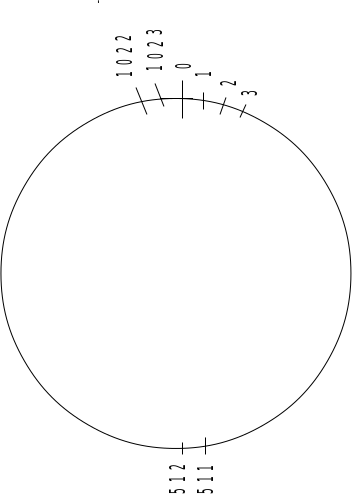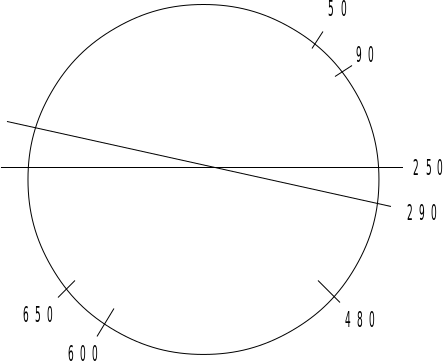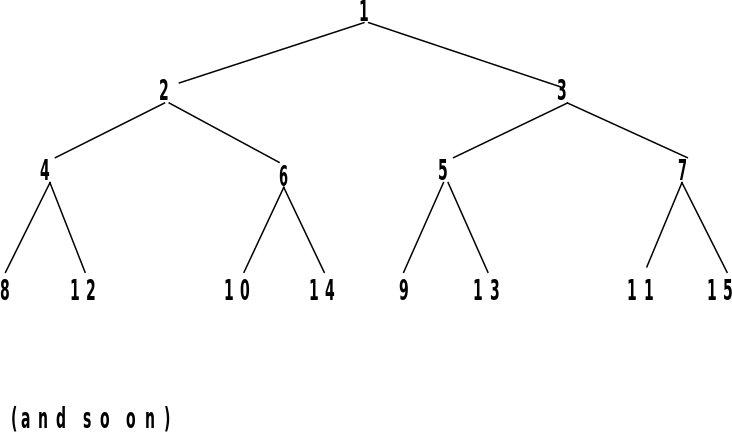## J.2 General principle

04.083GPPMobile radio interface Layer 3 specificationRelease 1998TS

The encoding algorithm is based on a recursive dichotomy of both the range (i.e. the set of values that are possible) and the subset (the values to encode).

The dichotomy is best understood if the range is seen as a circle. For instance, for the 1023 range:Figure J.1: Circular arrangement of 0..1023

The dichotomy consists in finding a value in the subset such that the diameter determined by this value splits the subset in two equal or nearly equal subsets. In the following case, we see that value 290 is acceptable (the two subsets have 3 elements), when value 250 is not acceptable (the two subsets have 4 and 2 elements):Figure J.2: Example of dichotomy

The pivot value is part of the information field, then the two subsets are renumbered and the same algorithm is applied again on each of them. Because the range is halved at each step, the number of bits needed to encode a pivot value is 1 bit less than the number of bits needed to encode the parent pivot value.

The convention is that if the number of values is even, the left subset (that is to say the values that can be expressed as the pivot value minus some integer between 1 and half the range) will have 1 element more than the right subset.

At each step the subset is numbered from 0 to the range minus 1. The coding in the information field of the pivot value is its value as renumbered, plus 1. Value 0 is reserved to indicate no element.

The order of appearance in the information field of the successive pivot values is particular. If we present the values as organized as a tree, with the left child being the pivot of the left subset and the right child the pivot of the right subset, the order of appearance is given by the following tree:Figure J.3

This order has been chosen so that:

a) whatever the number N of elements in the set, the meaningful nodes are the first N and the value for all nodes from N+1 on are null (if sent);

b) the tree and all subtrees are balanced.

Important properties of these trees are used in the algorithms (with generation 1 corresponding to the root):

Generation g contains 2g-1 nodes, and their indices are 2g-1 to 2g-1;

For generation g, nodes 2g-1 to 2g-1+2g-2-1 are left children, the others are right children;

If node k belongs to generation g, its left child is node k + 2g-1 , and its right child is k + 2g;

Reciprocally, if k is a left child from generation g, its parent node is node k – 2g-2, and if k is a right child of generation g, its parent is node k – 2g-1.